### 10.2. Other rules for the annotation of numbers and numerals

#### 10.2.1. Competition of a numeral with the attributive function and one with the meaning of a "container"

If a counted object is modified by two (or more) numerals in coordination or apposition, one of which should be assigned the functor `RSTR` (following the rules in Section 10.1.1, "Numerals with the role of an attribute (`RSTR`)") and the other one has the meaning of a container (see Section 10.1.2, "Numerals with the meaning of a "container""), then, both (all) nodes representing the numerals depend on the node for the counted object. Both (all) nodes get the `RSTR` functor.

Examples:

Tyč je dlouhá čtyři.`RSTR` a půl.`RSTR` metru. (=The pole is four and a half meters long) Fig. 8.188

Pracují už dvě.`RSTR` a čtvrt.`RSTR` hodiny. (=They have already worked for two and a quarter hours)

Mám tisíc.`RSTR` a jeden.`RSTR` důvod ti nevěřit. (=I have one thousand and one reasons not to believe you)

NB! This type is to be distinguished from the type pět milionů lidí (=five million people) where the numerals do not compete. See also Section 10.1.2, "Numerals with the meaning of a "container"".

Figure 8.188. Competition of a numeral with the attributive function and one with the meaning of a "container"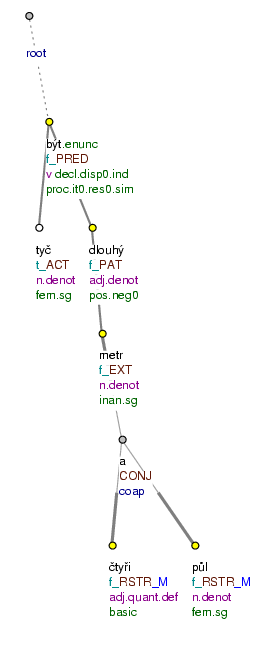Tyč je dlouhá čtyři a půl metru. (=lit. (The) pole is long four and half meter)

#### 10.2.2. Numeral expressions "hodně", "více", "dost", "moc", "málo", "méně", "stejně", "plno"

Adverbial meanings (see Section 10.1.4, "Numerals with adverbial meanings"). Numeral expressions hodně (=a lot), dost (=enough), moc (=much), málo (=little), plno (=plenty), stejně (=equally / to_the same_degree) (and their comparative forms více (=more), méně (=less)) have usually adverbial functions (they are mostly assigned the functor `EXT`).

Examples:

Hodně.`EXT` mě zajímalo, jak to funguje. (=I was interested a lot in knowing how it works) Fig. 8.189

Musel se chovat hodně.`EXT` opatrně (=He had to behave very cautiously).

Polévka je málo.`EXT` slaná (=The soup is not salty enough).

Attributive function (see Section 10.1.1, "Numerals with the role of an attribute (`RSTR`)"). When combined with a counted noun, the numerals hodně, dost, moc, málo, plno, stejně (and their comparative (and superlative) forms více, méně) have the adjectival function. The node representing a numerals like these depends on the node for the counted noun and has the `RSTR` functor. If the counted object (the governing noun) is not present at the surface level, a new node is inserted in its position in the tectogrammatical tree, following the rules in Section 12.1.2, "Ellipsis of the governing noun".

Examples:

Vrátili mu hodně.`RSTR` {`#EmpNoun`.`PAT`} . (=They returned him a lot) Fig. 8.190

Mám toho.`PAT` dost.`RSTR` (=I've got enough) (dost depends on toho)

Vydělá víc.`RSTR` {`#EmpNoun`.`PAT`} (=He earns more)

Vydělá stejně.`RSTR` {`#EmpNoun`.`PAT`} jako vloni. (=He is going to earn the same (money) as last year)

Dostal méně.`RSTR` {`#EmpNoun`.`PAT`} (=He got less)

NB! When the numerical expression is in the position of the Patient or Effect and agrees with another valency modification (this is a traditional predicative complement), when it is in the position of a predicative complement or the nominal part of a verbonominal predicate, no new node for the counted noun is inserted (see also Section 10.1.1, "Numerals with the role of an attribute (`RSTR`)").

Examples:

Studentů přišlo hodně.`COMPL` (=lit. Students came many) Fig. 8.191

To je hodně.`PAT` (=This is a lot) Fig. 8.192

Festivalových filmů existuje jen málo.`COMPL` (=lit. Festival movies exist only few)

Peněz mu vrátili hodně.`COMPL` (=lit. Money (they) him returned a lot)

Studentů přišlo více.`COMPL` (=lit. Students came more)

Děvčat je málo.`PAT` (=lit. Girls are few)

To je moc.`PAT` (=This is too much)

To je o důvod víc.`PAT` (=This is one reason more)

NB! A special rule is applied in comparative constructions in which quantities are compared (see Section 4.2.1, "Comparing quantities by means of the conjunction "než"").

Figure 8.189. The numeral hodně as an adverbial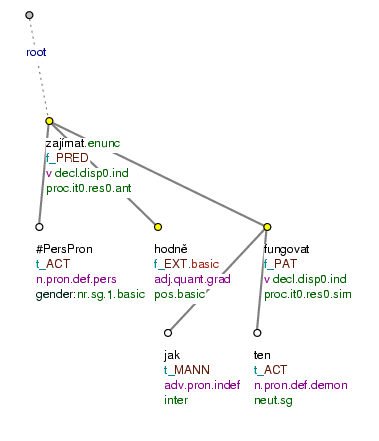Hodně mě zajímalo, jak to funguje. (=lit. A_lot me interested how it works)

Figure 8.190. The numeral hodně as an attribute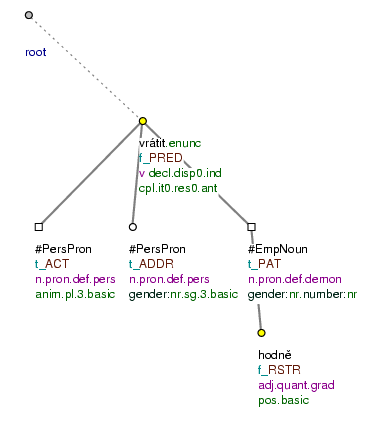Vrátili mu hodně. (=lit. (They) returned him a_lot)

Figure 8.191. The numeral hodně as a predicative complement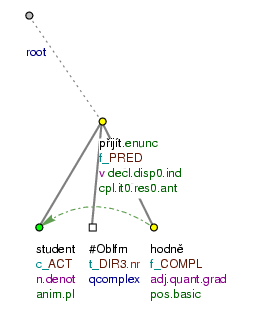Studentů přišlo hodně. (=lit. Of_students came a_lot)

Figure 8.192. The numeral hodně as the Patient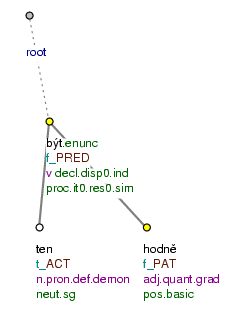To je hodně. (=lit. This is a_lot)

#### 10.2.3. Complex numerical expressions

##### 10.2.3.1. Type "sto čtyřicet tisíc lidí"

Complex numerical expressions of the type sto čtyřicet tisíc (=one hundred and forty thousand), dva miliony pět set tisíc (=two million five hundred thousand), třicet osm (=thirty-eight) are not assigned any inner structure:

• if a complex numerical expression contains one or more numerals with the meaning of a container, the numeral with the highest numerical value is analyzed as the governing node of the whole numerical expression. The governing node of a complex numerical expression is assigned a functor corresponding to its position in the structure. The nodes representing all the other parts of the numerical expression depend on the governing node (they are sisters w.r.t. each other) and are assigned the `RSTR` functor. The node representing the (possible) counted object has the `MAT` functor and depends on the governing node of the complex numerical expression.

Examples:

sto.`RSTR` čtyřicet.`RSTR` tisíc židů.`MAT` (=one hundred and forty thousand Jews) Fig. 8.193

Vydělal dva.`RSTR` tisíce.`PAT` čtyři.`RSTR` sta.`RSTR` pět.`RSTR` korun.`MAT` (=He earned two thousand four hundred and five crowns)

Žije zde jeden.`RSTR` milion.`ACT` pět.`RSTR` set.`RSTR` tisíc.`RSTR` lidí.`MAT` (=One million five hundred thousand people live here)

• if there is no numeral with the meaning of a container in the complex numerical expression, the governing node of the expression is the node for the counted object and all the other parts of the expression depend on the governing node (they are sister w.r.t. each other) and are assigned the `RSTR` functor.

Examples:

třicet.`RSTR` osm.`RSTR` žáků (=thirty-eight pupils) Fig. 8.194

Vydělal dvacet.`RSTR` pět.`RSTR` korun.`PAT` (=He earned twenty-five crowns)

Žije zde šedesát.`RSTR` dva.`RSTR` lidí.`ACT` (=Sixty-two people live here)

Numerals written in digits. If a numerical expression is written in digits, it is assigned a single node.

Examples:

Mám 38 234.`RSTR` korun.`PAT` (=I've got 38 234 crowns) Fig. 8.195

Mám 38 234.`PAT` (=I've got 38 234)

Vydělal 2 405.`RSTR` korun.`PAT` (=He earned 2 405 crowns)

Žije zde 1 500 000.`RSTR` lidí.`ACT` (=1 500 000 people live here)

Žije zde 1 500 000.`ACT` (=1 500 000 (people) live here)

Figure 8.193. Complex numerical expressions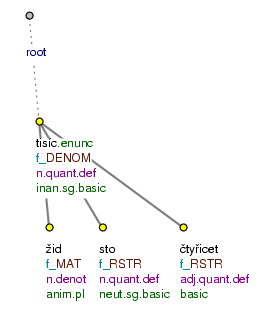sto čtyřicet tisíc židů (=lit. hundred forty thousand Jews)

Figure 8.194. Complex numerical expressions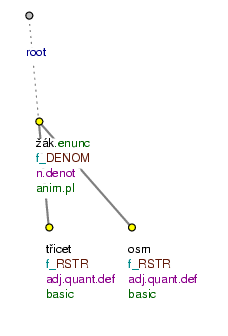třicet osm žáků (=lit. thirty eight pupils)

Figure 8.195. Complex numerical expressions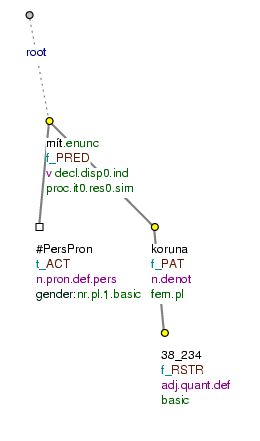Mám 38 234 korun. (=lit. (I) have 38 234 crowns)

##### 10.2.3.2. Type "tyč dlouhá 2 m 10 cm 4 mm"

Numerical expressions that are combinations of numerals and physical units, i.e. expressions of the type 2 m 10 cm 4 mm, 1 h 20 min are not assigned any inner structure. The governing node of the expression is the physical unit with the highest value. The governing node of the numerical expression is assigned a functor corresponding to its position in the structure. The nodes for the other physical units depend on the governing node (they are sisters w.r.t. each other) and get the `RSTR` functor; the nodes for the numerals depend on their respective nodes for the physical units and get the `RSTR` functor, too.

Examples:

Tyč je dlouhá 2 m.`EXT` 10 cm 4 mm. (=The pole is 2m 10cm 4mm long) Fig. 8.196

Film začíná ve 2 hod.`TWHEN` 35 min. (=The film begins at (lit.) 2h 35 min) (The following case is different: Film začíná ve 2 hod.`TWHEN` a 35 min.`TWHEN` (=The film begins at (lit.) 2h and 35 min))

Vážil 53 tun.`EXT` 15 kg. (=It weighed 53t 15 kg) (The following case is different: Vážil 53 tun.`EXT` a 15 kg.`EXT` (=It weighed 53t and 15kg))

NB! The rules as described above do not concern forms like 7: 30, which are analyzed (as paratactic structures) with the help of the `OPER` functor (see Section 11, "Mathematical operations and intervals"), and forms like dne 3. února roku 2003 (=on 3 February 2003), 3. 2. 2003 e.t.c., which are assigned inner structure (see Section 12.10, "Set expressions in journalism" and Section 11.3, "Mutual relation of two or more locative/directional or temporal modifications").

NB! Expressions of the type 40procentní (=40%.adj), 20metrový (=20m.adj) are represented by a single node with a t-lemma of the form: 40_procentní, 20_metrový (see Section 3.1, "Multi-word t-lemma").

Figure 8.196. Complex numerical expressions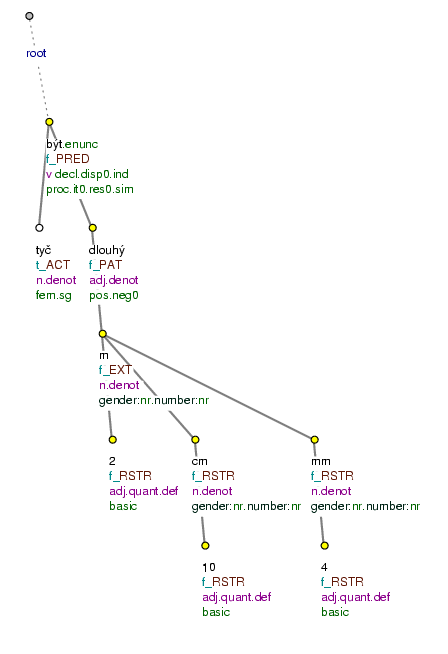Tyč je dlouhá 2 m 10 cm 4 mm. (=lit. Pole is long 2 m 10 cm 4 mm)

#### 10.2.4. Decimals and fractions

Decimals. Decimal numbers (written in digits) are represented as a single node and get a functor depending on their position in the structure.

Examples:

Výroba se zvýšila o 2,3.`RSTR` procenta. (=The production increased by 2.3 per cent)

Výstavu navštívilo 2,5.`RSTR` tisíc lidí. (=2.5 thousand people visited the exhibition)

Fractions. Fractions written in digits (with a slash) are analyzed with the help of the `OPER` functor (see Section 11, "Mathematical operations and intervals"), i.e. like paratactic structures. If a fraction is written in words, the rules described above are followed (especially Section 10.1.2, "Numerals with the meaning of a "container"").

Examples (fraction in digits):

1 / 3 vody odtekla. [`#Slash`.`OPER`] (=one third of the water flew off) Fig. 8.197

1 / 2 práce už je za námi. [`#Slash`.`OPER`] (=half of the work is over already)

Examples (fraction written in words):

1.`RSTR` třetina.`ACT` vody.`MAT` odtekla. (=one third of the water flew off) Fig. 8.198

1.`RSTR` polovina.`ACT` práce.`MAT` už je za námi. (=half of the work is over already)

Figure 8.197. Fractions written in digits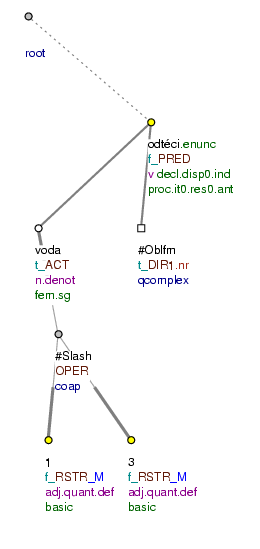1 / 3 vody odtekla. (=lit. 1/3 of_water flew_off)

Figure 8.198. Fractions written in words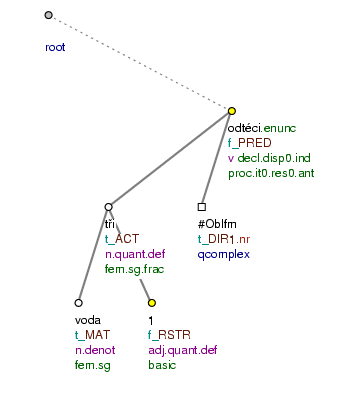1třetina vody odtekla. (=lit. 1 third of_water flew_off)